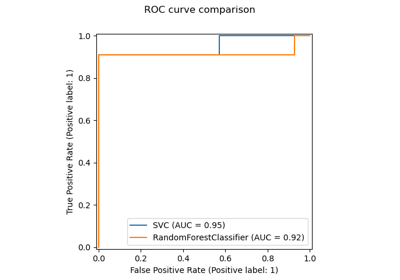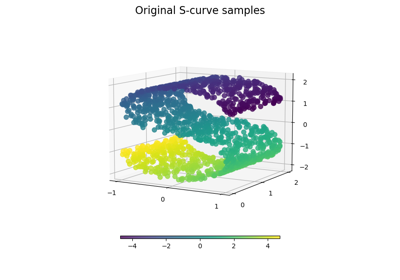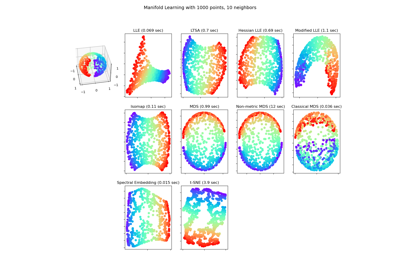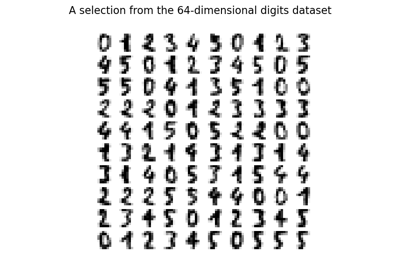# sklearn.manifold.Isomap¶

class sklearn.manifold.Isomap(*, n_neighbors=5, radius=None, n_components=2, eigen_solver='auto', tol=0, max_iter=None, path_method='auto', neighbors_algorithm='auto', n_jobs=None, metric='minkowski', p=2, metric_params=None)[source]

Isomap Embedding.

Non-linear dimensionality reduction through Isometric Mapping

Read more in the User Guide.

Parameters:
n_neighborsint or None, default=5

Number of neighbors to consider for each point. If n_neighbors is an int, then radius must be None.

Limiting distance of neighbors to return. If radius is a float, then n_neighbors must be set to None.

New in version 1.1.

n_componentsint, default=2

Number of coordinates for the manifold.

eigen_solver{‘auto’, ‘arpack’, ‘dense’}, default=’auto’

‘auto’ : Attempt to choose the most efficient solver for the given problem.

‘arpack’ : Use Arnoldi decomposition to find the eigenvalues and eigenvectors.

‘dense’ : Use a direct solver (i.e. LAPACK) for the eigenvalue decomposition.

tolfloat, default=0

Convergence tolerance passed to arpack or lobpcg. not used if eigen_solver == ‘dense’.

max_iterint, default=None

Maximum number of iterations for the arpack solver. not used if eigen_solver == ‘dense’.

path_method{‘auto’, ‘FW’, ‘D’}, default=’auto’

Method to use in finding shortest path.

‘auto’ : attempt to choose the best algorithm automatically.

‘FW’ : Floyd-Warshall algorithm.

‘D’ : Dijkstra’s algorithm.

neighbors_algorithm{‘auto’, ‘brute’, ‘kd_tree’, ‘ball_tree’}, default=’auto’

Algorithm to use for nearest neighbors search, passed to neighbors.NearestNeighbors instance.

n_jobsint or None, default=None

The number of parallel jobs to run. None means 1 unless in a joblib.parallel_backend context. -1 means using all processors. See Glossary for more details.

metricstr, or callable, default=”minkowski”

The metric to use when calculating distance between instances in a feature array. If metric is a string or callable, it must be one of the options allowed by sklearn.metrics.pairwise_distances for its metric parameter. If metric is “precomputed”, X is assumed to be a distance matrix and must be square. X may be a Glossary.

New in version 0.22.

pint, default=2

Parameter for the Minkowski metric from sklearn.metrics.pairwise.pairwise_distances. When p = 1, this is equivalent to using manhattan_distance (l1), and euclidean_distance (l2) for p = 2. For arbitrary p, minkowski_distance (l_p) is used.

New in version 0.22.

metric_paramsdict, default=None

Additional keyword arguments for the metric function.

New in version 0.22.

Attributes:
embedding_array-like, shape (n_samples, n_components)

Stores the embedding vectors.

kernel_pca_object

KernelPCA object used to implement the embedding.

nbrs_sklearn.neighbors.NearestNeighbors instance

Stores nearest neighbors instance, including BallTree or KDtree if applicable.

dist_matrix_array-like, shape (n_samples, n_samples)

Stores the geodesic distance matrix of training data.

n_features_in_int

Number of features seen during fit.

New in version 0.24.

feature_names_in_ndarray of shape (n_features_in_,)

Names of features seen during fit. Defined only when X has feature names that are all strings.

New in version 1.0.

sklearn.decomposition.PCA

Principal component analysis that is a linear dimensionality reduction method.

sklearn.decomposition.KernelPCA

Non-linear dimensionality reduction using kernels and PCA.

MDS

Manifold learning using multidimensional scaling.

TSNE

T-distributed Stochastic Neighbor Embedding.

LocallyLinearEmbedding

Manifold learning using Locally Linear Embedding.

SpectralEmbedding

Spectral embedding for non-linear dimensionality.

References



Tenenbaum, J.B.; De Silva, V.; & Langford, J.C. A global geometric framework for nonlinear dimensionality reduction. Science 290 (5500)

Examples

>>> from sklearn.datasets import load_digits
>>> from sklearn.manifold import Isomap
>>> X.shape
(1797, 64)
>>> embedding = Isomap(n_components=2)
>>> X_transformed = embedding.fit_transform(X[:100])
>>> X_transformed.shape
(100, 2)


Methods

 fit(X[, y]) Compute the embedding vectors for data X. fit_transform(X[, y]) Fit the model from data in X and transform X. get_feature_names_out([input_features]) Get output feature names for transformation. Get metadata routing of this object. get_params([deep]) Get parameters for this estimator. Compute the reconstruction error for the embedding. set_output(*[, transform]) Set output container. set_params(**params) Set the parameters of this estimator. Transform X.
fit(X, y=None)[source]

Compute the embedding vectors for data X.

Parameters:
X{array-like, sparse matrix, BallTree, KDTree, NearestNeighbors}

Sample data, shape = (n_samples, n_features), in the form of a numpy array, sparse matrix, precomputed tree, or NearestNeighbors object.

yIgnored

Not used, present for API consistency by convention.

Returns:
selfobject

Returns a fitted instance of self.

fit_transform(X, y=None)[source]

Fit the model from data in X and transform X.

Parameters:
X{array-like, sparse matrix, BallTree, KDTree}

Training vector, where n_samples is the number of samples and n_features is the number of features.

yIgnored

Not used, present for API consistency by convention.

Returns:
X_newarray-like, shape (n_samples, n_components)

X transformed in the new space.

get_feature_names_out(input_features=None)[source]

Get output feature names for transformation.

The feature names out will prefixed by the lowercased class name. For example, if the transformer outputs 3 features, then the feature names out are: ["class_name0", "class_name1", "class_name2"].

Parameters:
input_featuresarray-like of str or None, default=None

Only used to validate feature names with the names seen in fit.

Returns:
feature_names_outndarray of str objects

Transformed feature names.

Get metadata routing of this object.

Please check User Guide on how the routing mechanism works.

Returns:

A MetadataRequest encapsulating routing information.

get_params(deep=True)[source]

Get parameters for this estimator.

Parameters:
deepbool, default=True

If True, will return the parameters for this estimator and contained subobjects that are estimators.

Returns:
paramsdict

Parameter names mapped to their values.

reconstruction_error()[source]

Compute the reconstruction error for the embedding.

Returns:
reconstruction_errorfloat

Reconstruction error.

Notes

The cost function of an isomap embedding is

E = frobenius_norm[K(D) - K(D_fit)] / n_samples

Where D is the matrix of distances for the input data X, D_fit is the matrix of distances for the output embedding X_fit, and K is the isomap kernel:

K(D) = -0.5 * (I - 1/n_samples) * D^2 * (I - 1/n_samples)

set_output(*, transform=None)[source]

Set output container.

See Introducing the set_output API for an example on how to use the API.

Parameters:
transform{“default”, “pandas”}, default=None

Configure output of transform and fit_transform.

• "default": Default output format of a transformer

• "pandas": DataFrame output

• None: Transform configuration is unchanged

Returns:
selfestimator instance

Estimator instance.

set_params(**params)[source]

Set the parameters of this estimator.

The method works on simple estimators as well as on nested objects (such as Pipeline). The latter have parameters of the form <component>__<parameter> so that it’s possible to update each component of a nested object.

Parameters:
**paramsdict

Estimator parameters.

Returns:
selfestimator instance

Estimator instance.

transform(X)[source]

Transform X.

This is implemented by linking the points X into the graph of geodesic distances of the training data. First the n_neighbors nearest neighbors of X are found in the training data, and from these the shortest geodesic distances from each point in X to each point in the training data are computed in order to construct the kernel. The embedding of X is the projection of this kernel onto the embedding vectors of the training set.

Parameters:
X{array-like, sparse matrix}, shape (n_queries, n_features)

If neighbors_algorithm=’precomputed’, X is assumed to be a distance matrix or a sparse graph of shape (n_queries, n_samples_fit).

Returns:
X_newarray-like, shape (n_queries, n_components)

X transformed in the new space.

## Examples using sklearn.manifold.Isomap¶Release Highlights for scikit-learn 0.22

Release Highlights for scikit-learn 0.22Comparison of Manifold Learning methods

Comparison of Manifold Learning methodsManifold Learning methods on a severed sphere

Manifold Learning methods on a severed sphereManifold learning on handwritten digits: Locally Linear Embedding, Isomap…

Manifold learning on handwritten digits: Locally Linear Embedding, Isomap...